User:Tohline/Appendix/CGH/Overview

(Difference between revisions)
 Revision as of 21:07, 27 December 2017 (view source)Tohline (Talk | contribs) (Created page with ' =CGH: Philosophical Overview= {{LSU_HBook_header}} ==Propagation of…')← Older edit Revision as of 21:25, 27 December 2017 (view source)Tohline (Talk | contribs) (→CGH: Philosophical Overview)Newer edit → Line 2: Line 2: =CGH:  Philosophical Overview= =CGH:  Philosophical Overview= - {{LSU_HBook_header}} {{LSU_HBook_header}} - ==Propagation of Light== + ==Slit Diffraction== + ===Single Aperture=== +
+ + + + + + + +
Figure 1
[[File:Aperture3.gif|350px|Chapter1Fig1]]
+
+ As has been detailed in an [[User:Tohline/Appendix/CGH/ParallelApertures#One-Dimensional_Aperture|accompanying discussion]], we consider, first, the amplitude (and phase) of light that is incident at a location $~y_1$ on an image screen that is located a distance $~Z$ from a slit of width $~w = (Y_1 - Y_2) = 2c$.  The amplitude is given by the expression, +
+ + +
+ $~A(y_1)$ + + $~=$ + + $~\sum_j + a_j \biggl[ \cos\biggl(\frac{2\pi D_j}{\lambda} + \phi_j \biggr) + i \sin\biggl(\frac{2\pi D_j}{\lambda} + \phi_j \biggr) \biggr] + \, , +$ +
+ + + + + + + where, +
+ + +
+ $~D_j$ + + $~=$ + + $~ + L \biggl[1 - \frac{2y_1 Y_j}{L^2} + \frac{Y_j^2}{L^2} \biggr]^{1 / 2} \, , +$ +
+ + + + + + + and, +
+ +
+ $~L$ + + $~\equiv$ + + $~ + [Z^2 + y_1^2 ]^{1 / 2} \, . +$ +

CGH: Philosophical Overview

Slit Diffraction

Single Aperture

As has been detailed in an accompanying discussion, we consider, first, the amplitude (and phase) of light that is incident at a location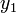$~y_1$ on an image screen that is located a distance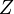$~Z$ from a slit of width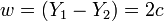$~w = (Y_1 - Y_2) = 2c$. The amplitude is given by the expression,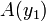$~A(y_1)$$~=$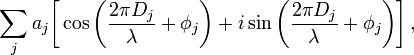$~\sum_j a_j \biggl[ \cos\biggl(\frac{2\pi D_j}{\lambda} + \phi_j \biggr) + i \sin\biggl(\frac{2\pi D_j}{\lambda} + \phi_j \biggr) \biggr] \, ,$

where,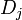$~D_j$$~=$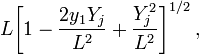$~ L \biggl[1 - \frac{2y_1 Y_j}{L^2} + \frac{Y_j^2}{L^2} \biggr]^{1 / 2} \, ,$

and,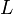$~L$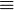$~\equiv$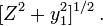$~ [Z^2 + y_1^2 ]^{1 / 2} \, .$